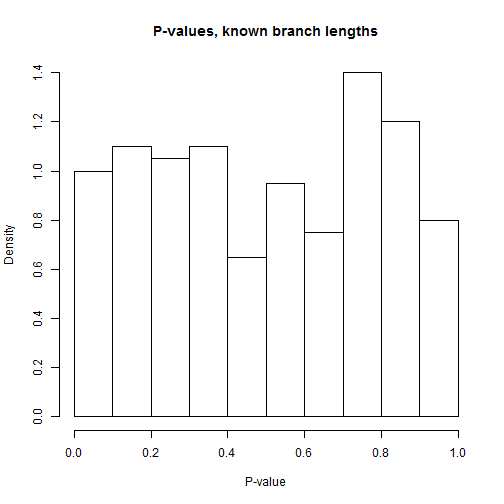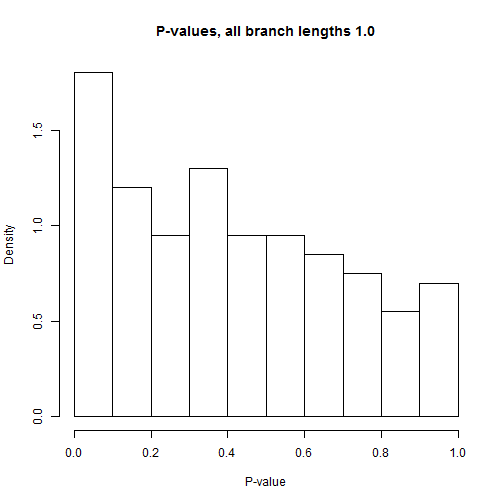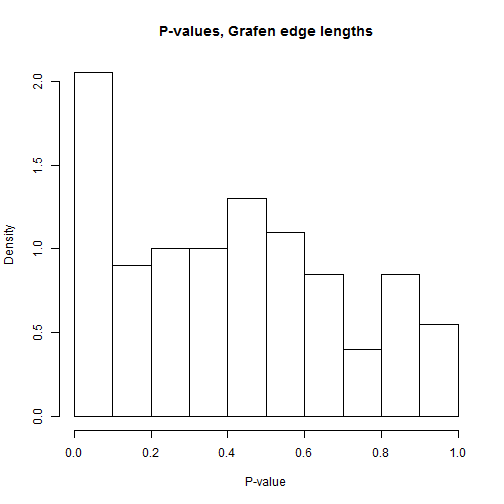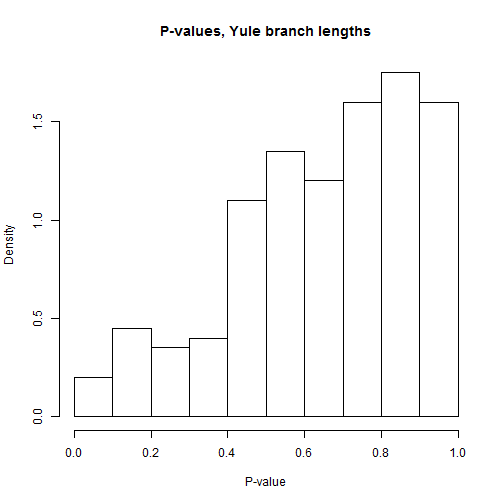## Tuesday, April 14, 2015

### Phylogenetic regression when branch lengths are unknown: A few different scenarios

In a recent R-sig-phylo discussion a user asked if it would be reasonable to use branches sampled assuming a Yule process in phylogenetic regression under conditions in which the branch lengths of the tree are unknown. Although I supplied a function designed to sample branching times on an arbitrary topology given this process, I could not answer the question of whether it was a good idea (or not) to use Yule process edge lengths when the topology was known, but branch lengths are not, even if it is reasonable to assume that the tree arose under a pure-birth process.

Here, I attempt to briefly explore that question.

First, my function to sample edges lengths under a Yule process (from last time):

``````yuleEdges<-function(tree,b=1,plot=TRUE,...){
ll<-rexp(n=Ntip(tree)-1,rate=2:Ntip(tree)*b)
tree\$edge.length<-rep(0,nrow(tree\$edge))
live.nodes<-Descendants(tree,Ntip(tree)+1,"children")
tips<-vector()
for(i in 1:length(ll)){
tips<-c(tips,live.nodes[live.nodes<=Ntip(tree)])
live.nodes<-setdiff(live.nodes,tips)
ii<-which(tree\$edge[,2]%in%c(live.nodes,tips))
tree\$edge.length[ii]<-tree\$edge.length[ii]+ll[i]
node<-if(length(live.nodes)<=1) live.nodes else
sample(live.nodes,1) ## choose one node
live.nodes<-c(setdiff(live.nodes,node),
Descendants(tree,node,"children"))
if(plot) plotTree(tree,...)
}
tree
}
``````

Now, let's simulate some data. First, let's try uncorrelated data. We can thus explore bias & variance in the estimate of the contrasts/PGLS regression slope (it should be zero), as well as type I error.

``````## load libraries
library(phytools)
library(phangorn)
library(ape)
## simulate 200 pure-birth trees:
trees<-ttrees<-pbtree(n=50,scale=1,nsim=200)
## ttrees contains the trees with their original branch lengths
## we'll perform manipulations on trees
foo<-function(tree){
obj<-fastBM(tree,nsim=2)
colnames(obj)<-c("x","y")
as.data.frame(obj)
}
xy<-lapply(ttrees,foo)
``````

Next, as a control for our experiment, let's fit contrasts regressions (PGLS,here) to each of these, using the known, true branch lengths. We'll just pull out the slope, β1 and the P-value for each regression:

``````library(nlme)
fit.model<-function(tree,data){
data\$v<-diag(vcv.phylo(tree))
fit<-gls(y~x,data=data,correlation=corBrownian(1,tree),
weights=varFixed(~v))
setNames(c(coefficients(fit),anova(fit)\$"p-value"),
c("beta","p-value"))
}
fit.true<-t(mapply(fit.model,trees,xy))
mean(fit.true[,1]) ## should be zero
``````
``````##  -0.005196606
``````
``````## should be uniform on [0,1]
hist(fit.true[,2],freq=FALSE,xlab="P-value",
main="P-values, known branch lengths")
````````````mean(fit.true[,2]<=0.05) ## should be about 0.05
``````
``````##  0.035
``````

OK, now imagine we are in a situation without branch lengths. We're going to consider a few different possibilities:

(1) All branch lengths set equal to 1.0.

(2) Grafen's (1989) branch lengths.

(3) Branch lengths randomly sampled under a Yule process.

First (1), setting all branch lengths to zero:

``````foo<-function(tree){
tree\$edge.length<-rep(1,nrow(tree\$edge))
tree
}
trees<-lapply(ttrees,foo)
class(trees)<-"multiPhylo"
fit.equal<-t(mapply(fit.model,trees,xy))
mean(fit.equal[,1]) ## should be zero
``````
``````##  -0.006076158
``````
``````## should be uniform on [0,1]
hist(fit.equal[,2],freq=FALSE,xlab="P-value",
main="P-values, all branch lengths 1.0")
````````````mean(fit.equal[,2]<=0.05) ## should be 0.05
``````
``````##  0.125
``````

Now (2), using Grafen's (1989) branch lengths:

``````trees<-lapply(ttrees,compute.brlen)
class(trees)<-"multiPhylo"
fit.grafen<-t(mapply(fit.model,trees,xy))
mean(fit.grafen[,1]) ## should be zero
``````
``````##  -0.007811392
``````
``````## should be uniform on [0,1]
par(mar=c(5.1,4.1,4.1,2.1))
hist(fit.grafen[,2],freq=FALSE,xlab="P-value",
main="P-values, Grafen edge lengths")
````````````mean(fit.grafen[,2]<=0.05) ## should be 0.05
``````
``````##  0.125
``````

Finally, (3), branch lengths sampled under a Yule process. Now for this, rather than using one set of branch lengths, for each tree we should simulate a set of branch lengths and then average our inference over this set. This is logical, because any individual set of branch lengths will be wrong, but perhaps by computing the variance among our estimated parameter and adding it to the mean variance of each estimate (under the law of total variance) should give us the correct variance of our estimator. Let's do this:

``````## this function simulates 100 sets of edge lengths, fits the model
## to each of them using PGLS, and extracts the coefficient & variance
## then averages the variance across trees, adds this to the variance
## among trees, and computes a p-value for the parameter from the
## t-distribution
yuleApply<-function(tree,data,nrep=100){
trees<-replicate(nrep,yuleEdges(tree,plot=FALSE),simplify=FALSE)
class(trees)<-"multiPhylo"
gls.fit<-function(tree,data){
obj<-gls(y~x,data=data,correlation=corBrownian(1,tree))
setNames(c(coefficients(obj),obj\$varBeta[2,2]),
c("beta","varBeta"))
}
fit<-t(sapply(trees,gls.fit,data=data))
b<-mean(fit[,"beta"])
v<-var(fit[,"beta"])+mean(fit[,"varBeta"])
p<-2*pt(abs(b/sqrt(v)),df=Ntip(tree)-1,lower.tail=FALSE)
setNames(c(b,v,p),c("beta","varBeta","p-value"))
}
fit.yule<-t(mapply(yuleApply,ttrees,xy))
mean(fit.yule[,1]) ## should be zero
``````
``````##  -0.03133003
``````
``````## should be uniform on [0,1]
hist(fit.yule[,3],freq=FALSE,xlab="P-value",
main="P-values, Yule branch lengths")
````````````mean(fit.yule[,3]<=0.05) ## should be 0.05
``````
``````##  0
``````

So, unless I messed something up here, it looks as though simulating edge lengths under a Yule process, though unbiased, is excessively conservative.

Next, let's try the situation in which x and y are genuinely correlated. Again, we start with the data generation, say with β1 = 0.4:

``````foo<-function(tree,beta,vare){
x<-fastBM(tree)
e<-fastBM(tree,sig2=vare)
y<-beta*x+e
data.frame(x,y)
}
xy<-lapply(ttrees,foo,beta=0.4,vare=1.2)
``````

First, with the true edge lengths:

``````fit.true<-t(mapply(fit.model,trees,xy))
mean(fit.true[,1]) ## should be 0.4
``````
``````##  0.3856536
``````
``````## this is power now, rather than type I error
mean(fit.true[,2]<=0.05)
``````
``````##  0.615
``````

Now all edge lengths to 1.0:

``````foo<-function(tree){
tree\$edge.length<-rep(1,nrow(tree\$edge))
tree
}
trees<-lapply(ttrees,foo)
class(trees)<-"multiPhylo"
fit.equal<-t(mapply(fit.model,trees,xy))
mean(fit.equal[,1]) ## should be 0.4
``````
``````##  0.3946721
``````
``````mean(fit.equal[,2]<=0.05) ## power
``````
``````##  0.605
``````

Now Grafen's edge lengths with `compute.brlen`:

``````trees<-lapply(ttrees,compute.brlen)
class(trees)<-"multiPhylo"
fit.grafen<-t(mapply(fit.model,trees,xy))
mean(fit.grafen[,1]) ## should be 0.4
``````
``````##  0.3856536
``````
``````mean(fit.grafen[,2]<=0.05) ## power
``````
``````##  0.615
``````

Now, branching times sampled under a Yule process, as before:

``````fit.yule<-t(mapply(yuleApply,ttrees,xy))
mean(fit.yule[,1]) ## should be 0.4
``````
``````##  0.3870531
``````
``````mean(fit.yule[,3]<=0.05) ## power
``````
``````##  0.145
``````

So, there you have it. Although it would seem to be the case that sampling branching times under which the known true branches arose, a Yule process, would be a good strategy when the branch lengths are unknown - this has the effect of leading to a type I error rate considerably below the nominal rate, as well as to low power relative to other kinds of arbitrary branch lengths. Who knew!

1.I tried something very similar on a dataset I was working on and also got the strong impression that using simulated Yule edge lengths was highly conservative and not very powerful. It's nice to see a proper assessment of this, even a quick one, and that it confirms my suspicions.

I wonder if there is some scope to develop such a method when some node heights are known. This is often the case when you have some dating information but none for the rest of an existing topology. Essentially, this would be similar to the BLADJ function in phylocom in that it uses what information is available, but rather than evenly spacing nodes between 'known' points it would simulate these from a Yule process. Perhaps such incorporation of some information on node heights would lead to reasonable performance of the method?

2.This is pretty interesting, but why branch-lengths from a Yule model? Given that the individual asking on R-Sig-Phylo noted he intended to add some extinct fossil taxa to his (apparently currently ultrametric) tree, why not a birth-death model?

1.It's a little bit more technically difficult to sample the waiting times with birth & death and a reconstructed phylogeny. Then we also have to keep track of whether each event is a speciation or extinction because that affects the waiting time to the next event. That said, it is possible. In addition after writing code to sample edge lengths in this way I became more interested in whether or not this was a good idea for PCMs - even when the model for the edge lengths and the model under which the true tree arose are the same (in this case, a Yule process).

Note: due to the very large amount of spam, all comments are now automatically submitted for moderation.